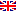Scheda programma d'esame
MATEMATICA DISCRETA E ALGEBRA LINEARE
ALESSANDRO BERARDUCCI
CdSINFORMATICA
Codice585AA
CFU12
PeriodoSecondo semestre

ModuliSettoreTipoOreDocente/i
ALGEBRA LINEAREMAT/03LEZIONI48
 ALESSANDRO BERARDUCCI unimap
MATEMATICA DISCRETAMAT/02LEZIONI48
 ALESSANDRO BERARDUCCI unimapEsporta in pdf
Obiettivi di apprendimento
Learning outcomes
Conoscenze

Vedere la pagina in Inglese selezionando il bottone corrispondente nel menù.

Knowledge

The student who successfully completes the course will be familiar with induction, simple counting and linear recurrence problems, modular arithmetic, vector spaces, bases and linear independence, determinants, linear maps. They will be able to solve on their own simple problems in these fields and will have developed an intuitive vision of the algebraic and geometric aspects of these topics. They will be able to provide proofs of the basic theoretical results seen in the lectures.

Assessment criteria of knowledge

The written exam contains (proof- or computation-based) exercises. Full detail on their solution is required, not only a final result. The oral exam includes theorem proofs and/or other exercises.

Methods:

• Final oral exam
• Final written exam

Further information:
Final written exam (or 2 x "compitini") and oral exam.

Teaching methods

Delivery: face to face

Learning activities:

• attending lectures
• individual study

Teaching methods:

• Lectures
Programma (contenuti dell'insegnamento)

Vedere la pagina in Inglese selezionando il relativo bottone nel menù.

Syllabus

Induction. Sequences and linear recurrences.

Divisibility, prime numbers, greatest common divisor, Bézout identity, congruences and modular arithmetic, inverses modulo n, Chinese Remainder theorem, Fermat's little theorem, Euler's phi function, RSA encryption algorithm.

Binomial coefficients, Newton's binomial formula, elementary combinatorics, inclusion-exclusion principle.

Polynomials. Euclidean division of polynomials. polynomial factorization. Complex roots.  Irreducible polynomials over the real numbers.

Linear Systems. Matrices. Gauss elimination. Echelon form. Matrix multiplication. Elementary matrices. Inverse matrix.

Vector spaces, linear dependence, dimension and basis.  Subspaces. Sum and intersection of subspaces.

Linear applications and associated matrices.  Kernel and image of a linear map. Rank.    Determinants.    Eigenvalues and eigenvectors.  Characteristic polynomial of an endomorphism.   Diagonalizability.

Orthogonality. Gram-Schmidt orthogonalization.

Bibliography

No mandatory reading. Suggested books and resources for individual study include:

* The online lecture notes provided by the teacher at http://people.dm.unipi.it/berardu/Didattica/2016-17MDAL/MDAL2016-17.html

* L. Childs, ALGEBRA, un'introduzione concreta, ETS Editrice. For the part "induction and arithmetic".

* M. Abate, /Algebra Lineare/, McGraw-Hill. * S. Abeasis, /Elementi di Algebra Lineare e Geometria/, Zanichelli.

* G. Strang, /Introduction to Linear Algebra/, Wellesley Cambridge, or his video lectures on http://ocw.mit.edu (both in English).

(not all of these books are necessary of course; an abundance of sources is listed for the students' benefit, but one or two of these books/resources will cover all the required material).

Non-attending students info

See http://people.dm.unipi.it/berardu/Didattica/2016-17MDAL/MDAL2016-17.html

Assessment methods

See http://people.dm.unipi.it/berardu/Didattica/2016-17MDAL/MDAL2016-17.html